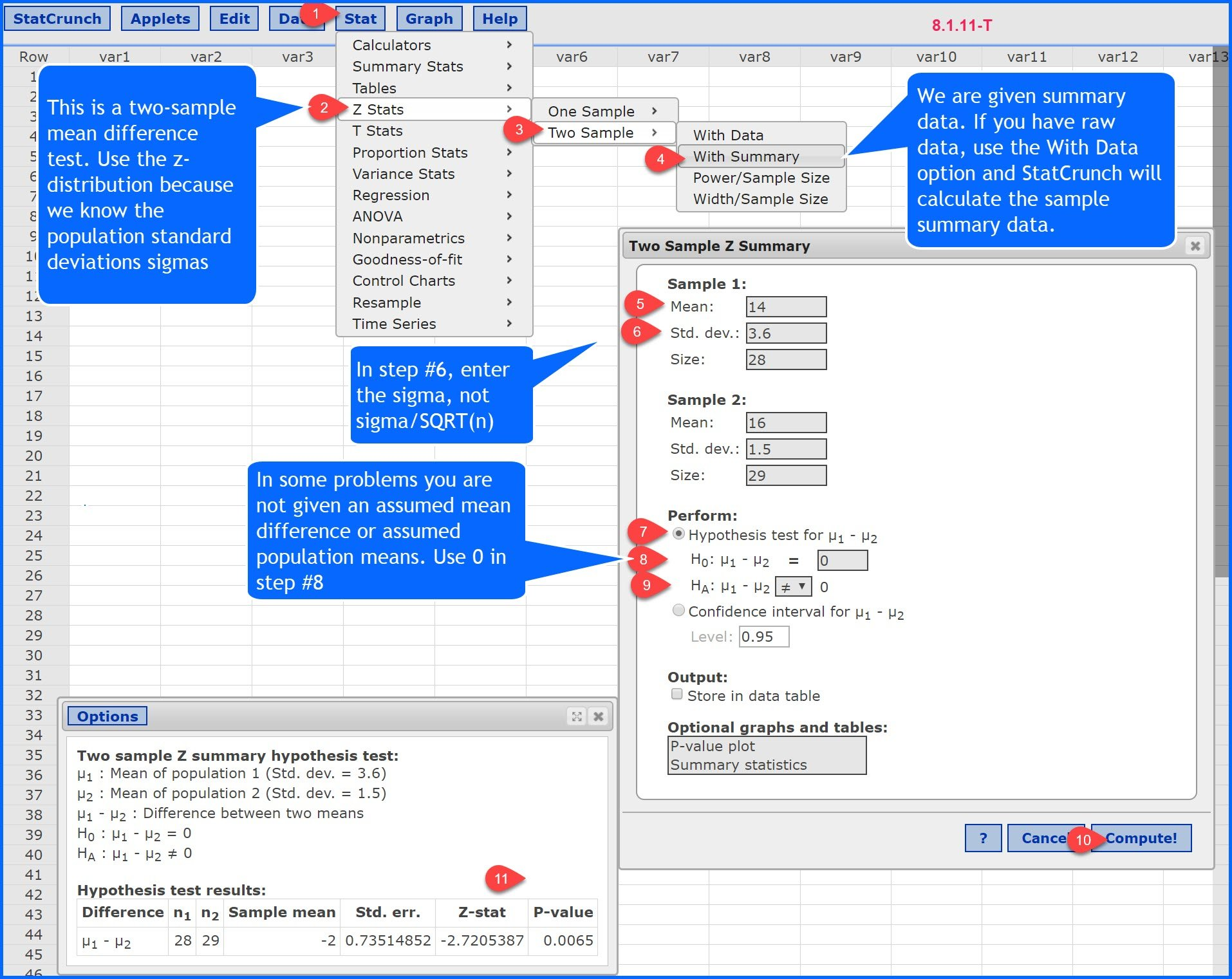Select Page

Question: I have hit the wall on a simple problem. I seem to be hitting these more often now.
At any rate. 8.1.11, the difference between two means hypothesis test standardized test statistic requires µ1 – µ2. So, how does one calculate this when we are never given a value for μ? I used 0 for the values, and came out with the WRONG answer. Request a nudge in the right direction.

Answer: James, for most of our mean difference problems, we will not be given the assumed population means or the mean difference. If not, you use 0 for the mean difference. In your problem, you are told that μ1 = μ2, so the mean difference  μ1 – μ2, is 0.

Here is the StatCrunch solution with slightly different summary data: